Numeracy Skills Assessment Paper
This assessment paper give examples of the basic literacy and language skill needed to attend a
TCS NDT course. It is not intended as a grading tool. It is to be used by students to determine if
they have the specific literacy and language to handle the course.
Email address *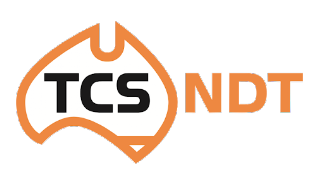Calculate the following equation.
1 point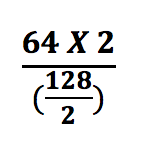How many millimetres in a metre?
1 point
Find the value of X from the equation:
1 point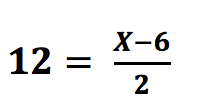Which Standard International prefix denotes the value One Thousand (1000)?
1 point
Using the values given, calculate the equation. D = 24, S = 2
1 point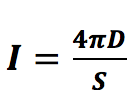A ratio of 75% water and 25% soap is required. If the volume of water is 100mL, how much soap is needed in order to achieve the desired ratio?
1 point
It takes 20 minutes to prepare a component for inspection, 1 ½ hours to conduct the inspection and half an hour to write the report. How much time in total will it take to perform the complete inspection and write the reports for two components?
1 point
The picture shows a defect located on a weld toe which has a start position of 98mm from the datum edge (0) and finishes at 128mm from the datum edge (0). What is the length of the defect?
1 point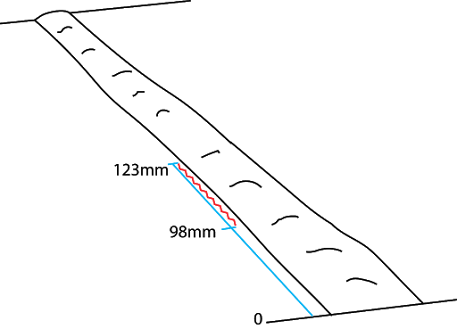This form was created inside of TCS NDT. Report Abuse - Terms of Service